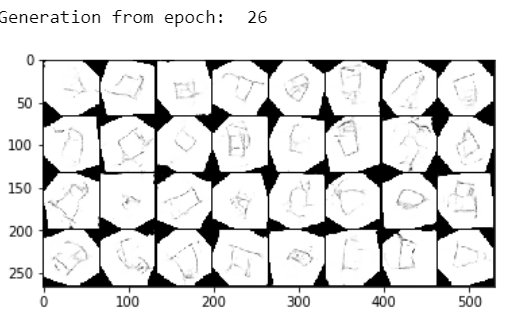# How do I fill the black or "blank" corners after I use rotation data augmentation?

``````transform = transforms.Compose([
transforms.Resize(64),
transforms.RandomRotation(360, resample=Image.BICUBIC),
transforms.ToTensor(),
transforms.Normalize(mean=(0.5, 0.5, 0.5), std=(0.5, 0.5, 0.5))
])
``````

After I call RandomRotation I get this imageIts screwing up the GAN I am trying to train.Whats the easiest way to fill the corners white?

2 Likes

some fill with nearest pixels, but that may be hard to implement. alternatively, you can increase the size (e.g., by using nearest pixels to fill in blank areas. this is easy because it is shifting in axes-aligned directions), and then rotate.

So let me get this straight I increase the white border size but not the content so when I rotate/crop I would still get to keep the corners? If so how do I increase the size is there a direct function you can call in pytorch?

Ideally you would want to do that in `dataset.__getitem__`, where you can operate on np arrays and/or PIL images. There are plenty of useful functions there.

1 Like